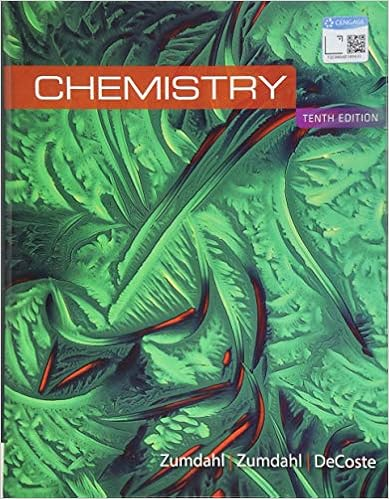# ch10.pdf - CHM2045 CH10 Homework Answer the quesions on the...

• 5

This preview shows page 1 - 2 out of 5 pages.

##### We have textbook solutions for you!
The document you are viewing contains questions related to this textbook.The document you are viewing contains questions related to this textbook.
Chapter 21 / Exercise 93
Chemistry
ZumdahlExpert Verified
CHM2045 CH10 HomeworkAnswer the quesions on the hardcopy first, then enter your answer keys on Canvas to get credit. To enter the answer keys, click"Assignments" on the left panel and then choose the appropriate chapter to work on.MULTIPLE CHOICE. Choose the one alternative that best completes the statement or answers the question.1.Determine the electron geometry (eg) and molecular geometry (mg) of SiBr4.1.A)eg =tetrahedral, mg =trigonal pyramidalB)eg =octahedral, mg =square planarC)eg =trigonal bipyramidal, mg =trigonal bipyramidalD)eg =tetrahedral, mg =bentE)eg =tetrahedral, mg =tetrahedral
2.What geometric arrangement of charge clouds is expected for an atom that has three charge clouds?
3.Using the VSEPR model, the molecular geometry of the central atom in NH4+is3.
4.Place the following in order of increasingX-Se-X bond angle, where X represents the outer atomsin each molecule.SeO2SeCl6SeF24.
6
2
6
25.Using the VSEPR model, the electron-domain geometry of the central atom in KrF2is5.A)linear.B)trigonal planar.C)tetrahedral.D)trigonal bipyramidal.E)octahedral.
##### We have textbook solutions for you!
The document you are viewing contains questions related to this textbook.The document you are viewing contains questions related to this textbook.
Chapter 21 / Exercise 93
Chemistry
ZumdahlExpert Verified
•••